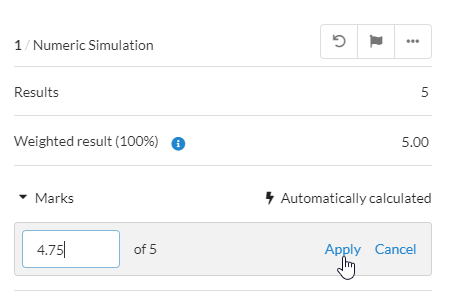# Question Type - Numerical simulation

Numerical Simulation question type allows you to create auto-marked maths questions with programmed parameterization and response outcomes to achieve randomization and more authentic assessment through real-world scenarios. Using randomization in tests ensures test integrity by giving each candidate a unique question.

Numerical Simulation utilizes a Program Model that enables simulations using an expressive programming language of Maxima which is based on Lisp.

# Numerical Simulation question type

## Introduction

Numerical Simulation is based on Numeric Entry, where the question is answered by typing a numeric value that is automatically scored. Numerical Simulation differs from Numeric Entry in that you are able to edit the Program Model that generates the correct and incorrect answers based on defined input variables that can be parameterised. The allocation of values to the Program Variables happens when the test is started and in this way, each test can have unique Program Variables. Numerical Simulation questions are automatically marked.

The “Pump Water” example used in this document is shared with permission from the creator - the UNSW School of Banking and Finance, University of New South Wales, Sydney, Australia.

## Program Model

The Program Model is a set of instructions that produce a set of input variables that the candidates use to calculate the results. The Program Model supports all the programming structures required to build programs of any complexity.

You can find more examples of what a Program Model might look like in our Help Center article, Numerical Simulation example guide.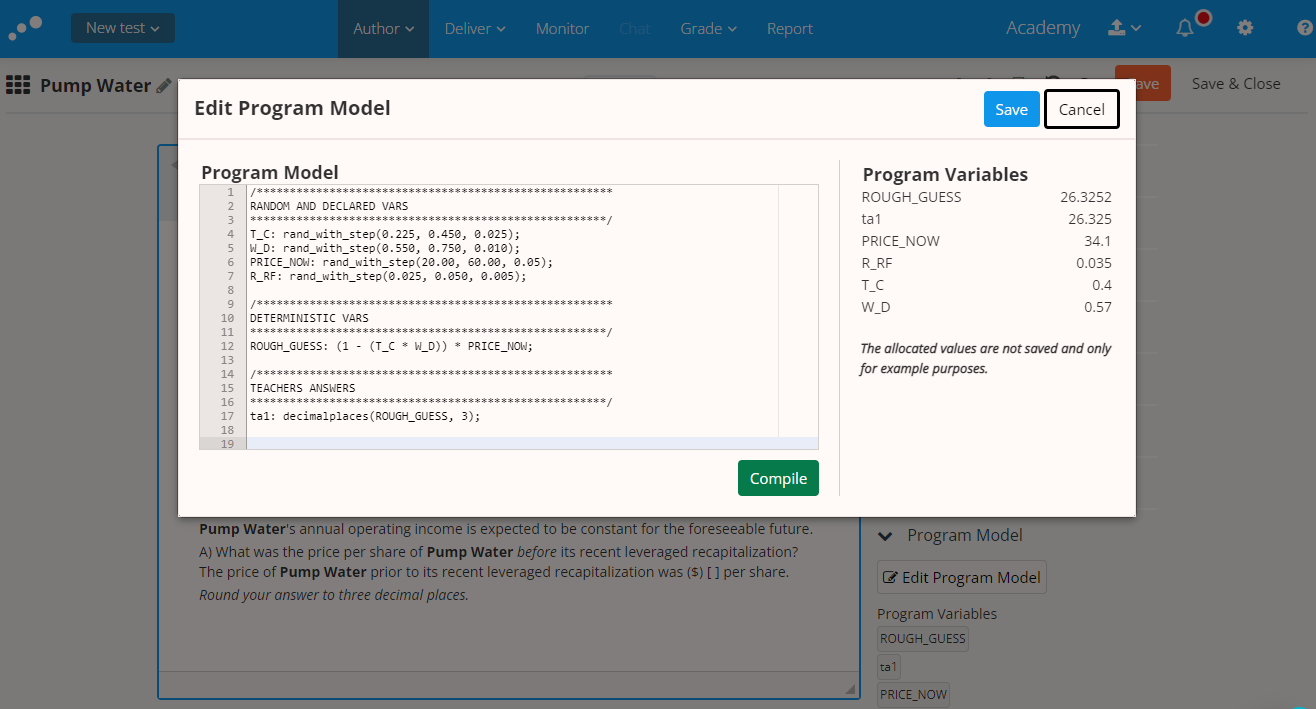## Insert Variables

Variables are created within your Program Model. When authoring a question, ensure that the wording allows for the insertion of input variables. The following variables are available:

• Program Variable: Variables compiled from the code.
• Candidate Input: Candidate answer field.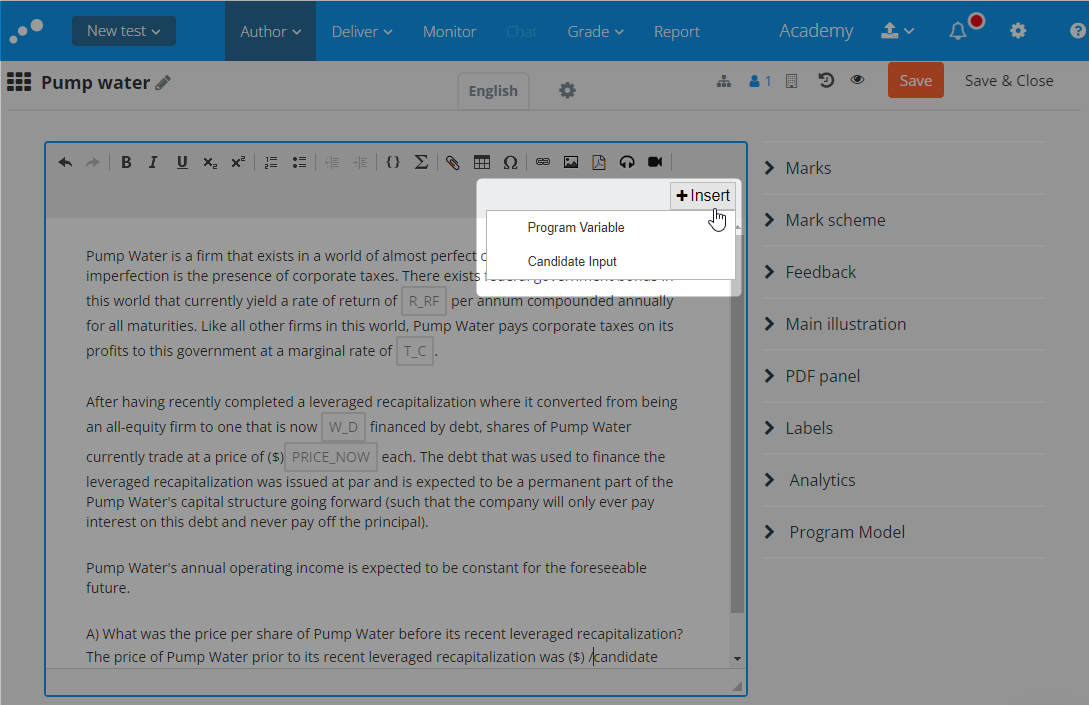# How to author a Numerical Simulation

## Create a new question

1. Select the Author module > Questions.
2. Click Create new.
3. Under Automatically marked, click Numerical Simulation.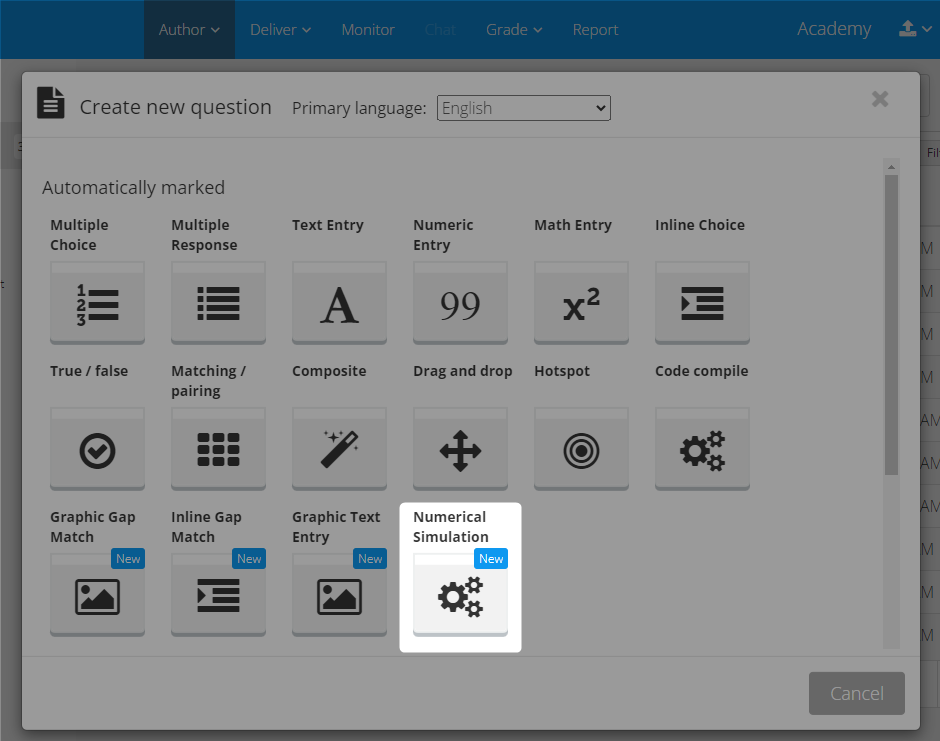## Edit and Compile Program Model

1. On the right-hand side, within the Question options, navigate to Program Model.
2. Select the Program Model drop-down > Edit program model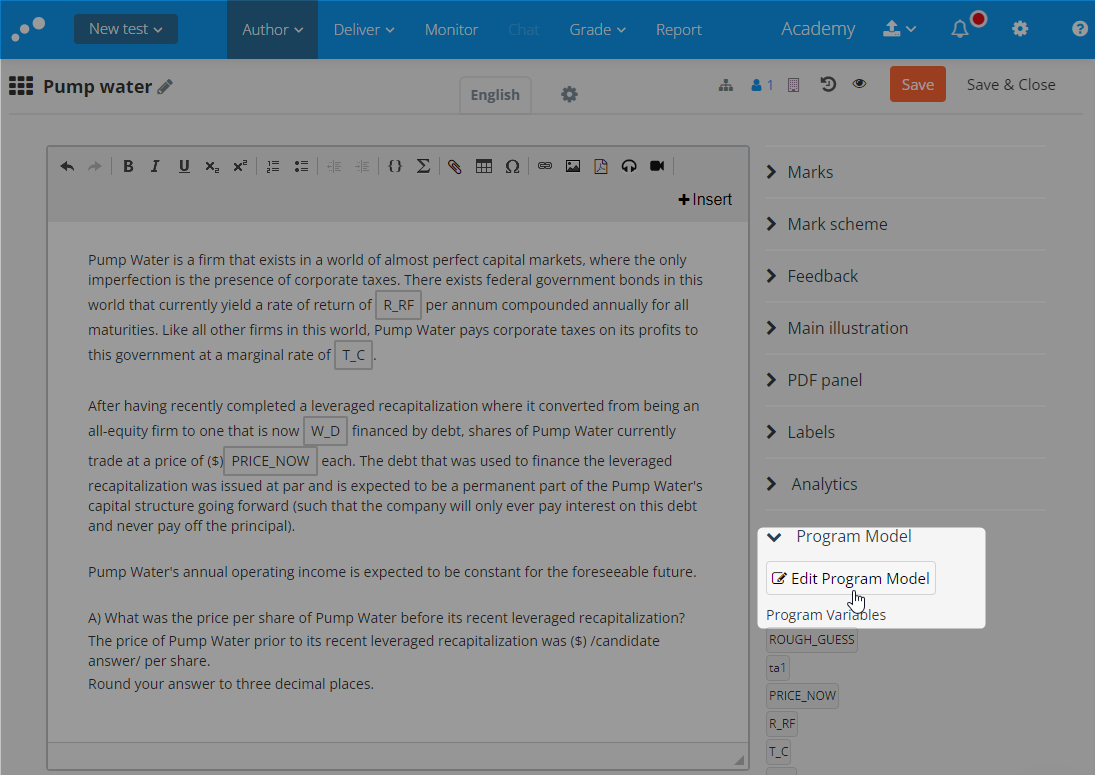### Compile the Program Model

1. Enter your code manually, or copy and paste text within the Program Model editor. Click Compile when you are finished.
• You are shown a sample of your Program Variables on the right-hand side once the compilation is successful. The allocated values are not saved and are only for example purposes.
2. To save your work, click Save.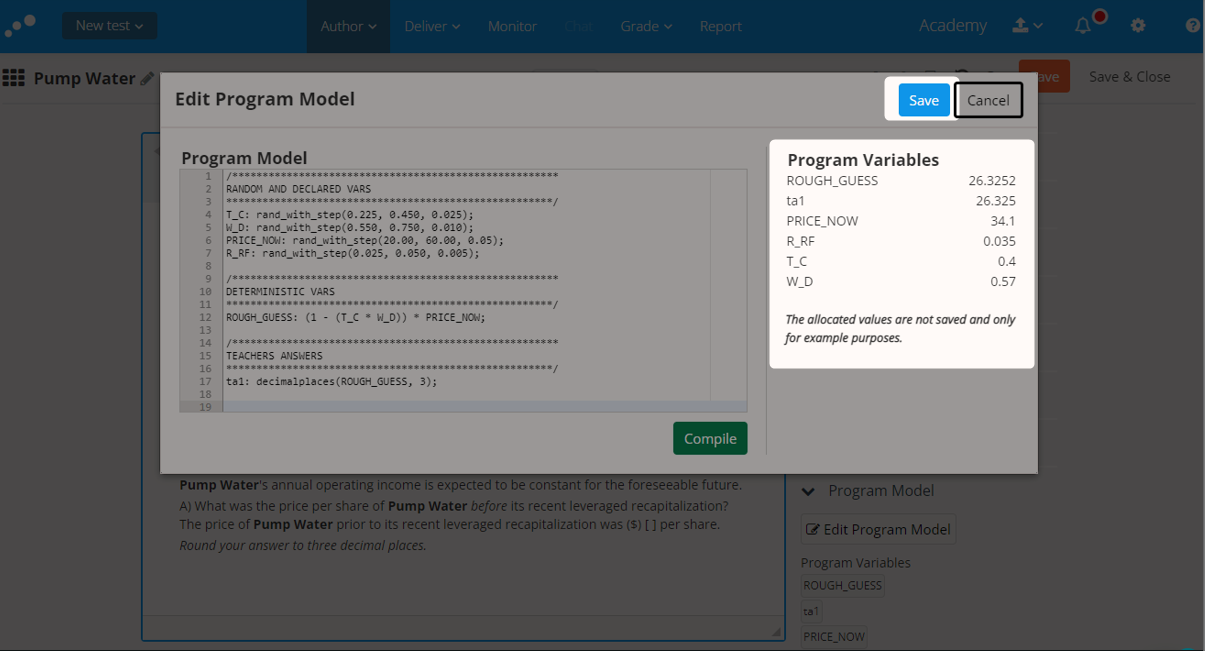### Enable Error carry forward variable

Where a question requires multiple responses from the candidate, and each response builds on a previous response to calculate the correct response, Error Carried Forward (ECF) enables candidates to receive partial marks where the specific answer is incorrect following from a previous error, but is mathematically correct.

In the example below we will first ask the candidate to double a number given by the variable [start_number], and then double this again in further responses. If they reach the incorrect answer in the first response, ECF will enable marking the subsequent answer as partially correct if they show the correct mathematical understanding, ie. doubling the value from the previous response.To set up ECF, we need to define ECF variables in the Program Model, and then set the ECF variable as a possible Response Outcomes for a given Response. In this way, we specify the formula to be used when determining if an incorrect answer is due to an incorrect answer in a previous part (error is carried forward), and specify the correct answer variable in that formula that should be replaced with the actual candidate response.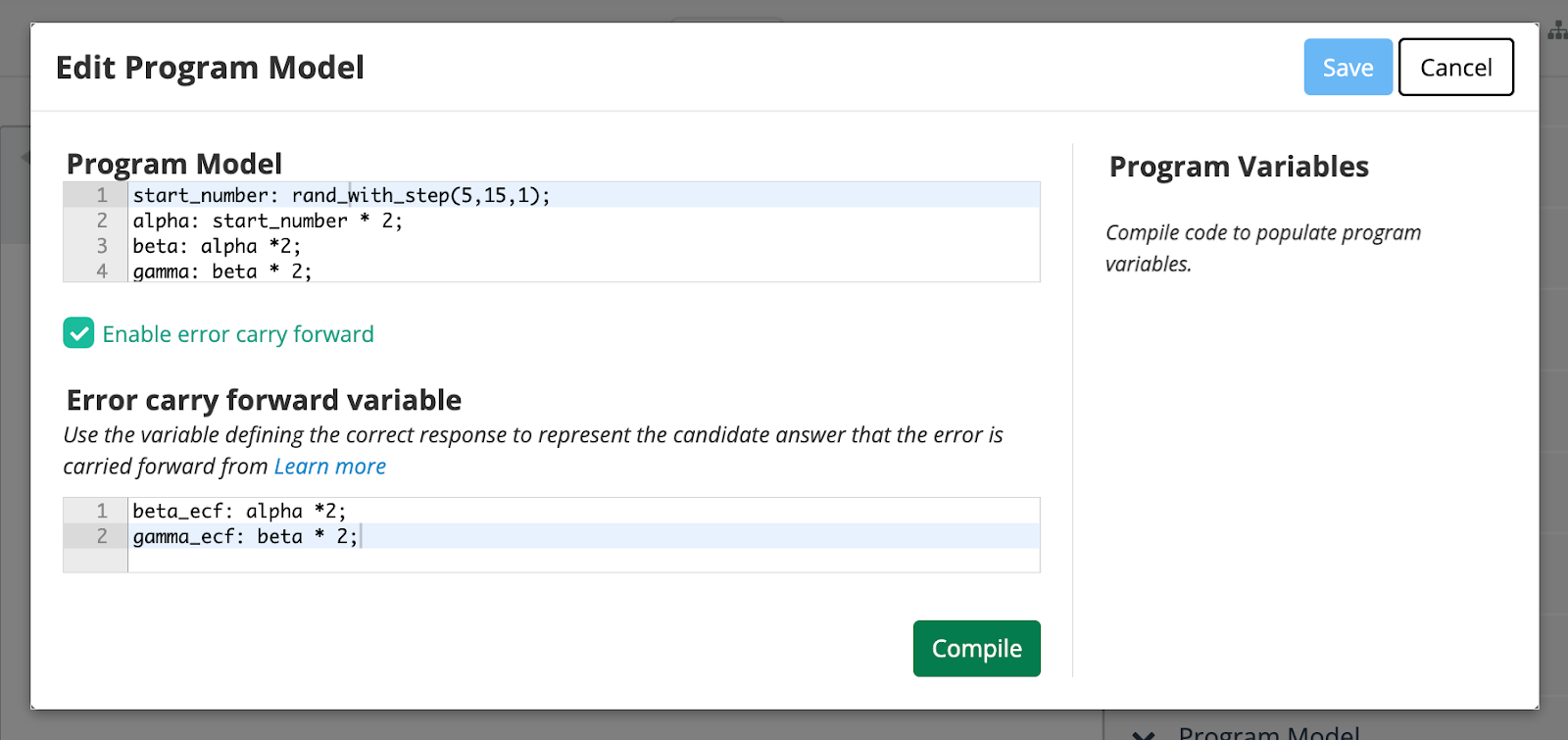Program Model with ECF enabled

Having enabled ECF, ECF variables can be defined. When defining an ECF variable, we need to include the variable that will be defined as correct in the earlier response that we want to enable an error to be carried forward from. When the system evaluates the ECF Response Outcome, this variable will be replaced with the actual candidate input to the response for which that variable is defined as correct.

In our example, “alpha” will be set as correct for RESPONSE-1´. “Beta” is calculated correctly by “alpha” * 2. When defining the ECF variable for “beta”, “alpha” is the variable that should be replaced by the value of the candidate input on that response when used in an ECF variable.

As “alpha” is defined as correct for RESPONSE-1,

The system evaluates, beta_ecf as

beta_ecf: RESPONSE-1 * 2;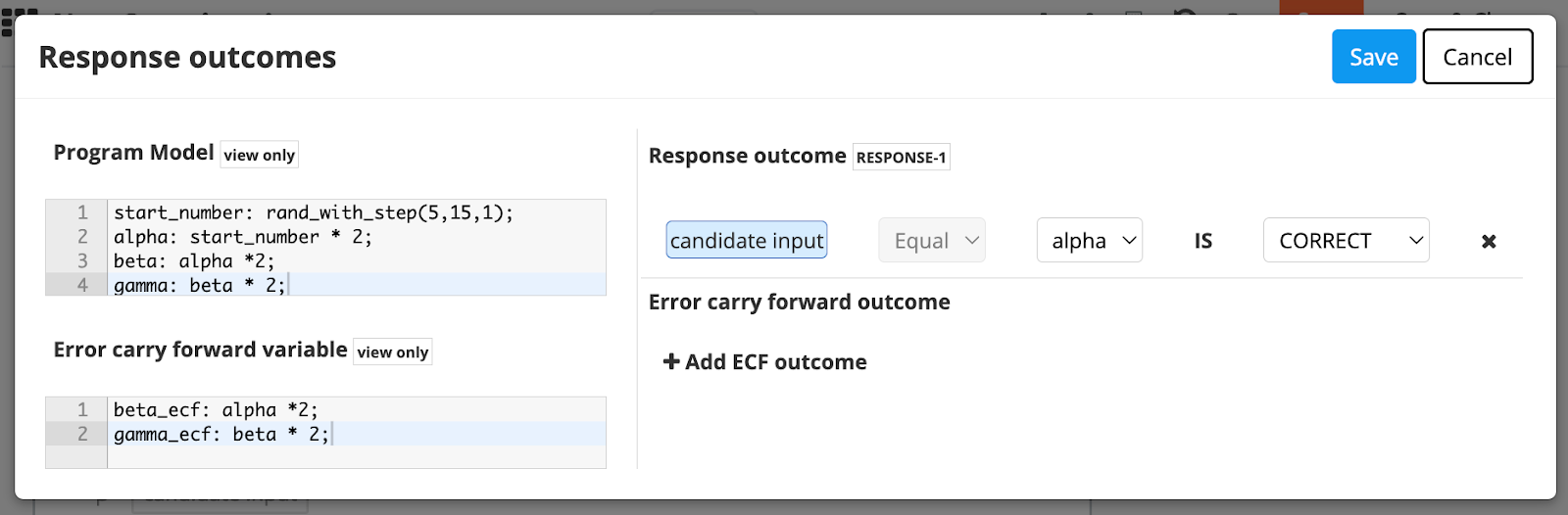Response Outcome RESPONSE-1 “alpha”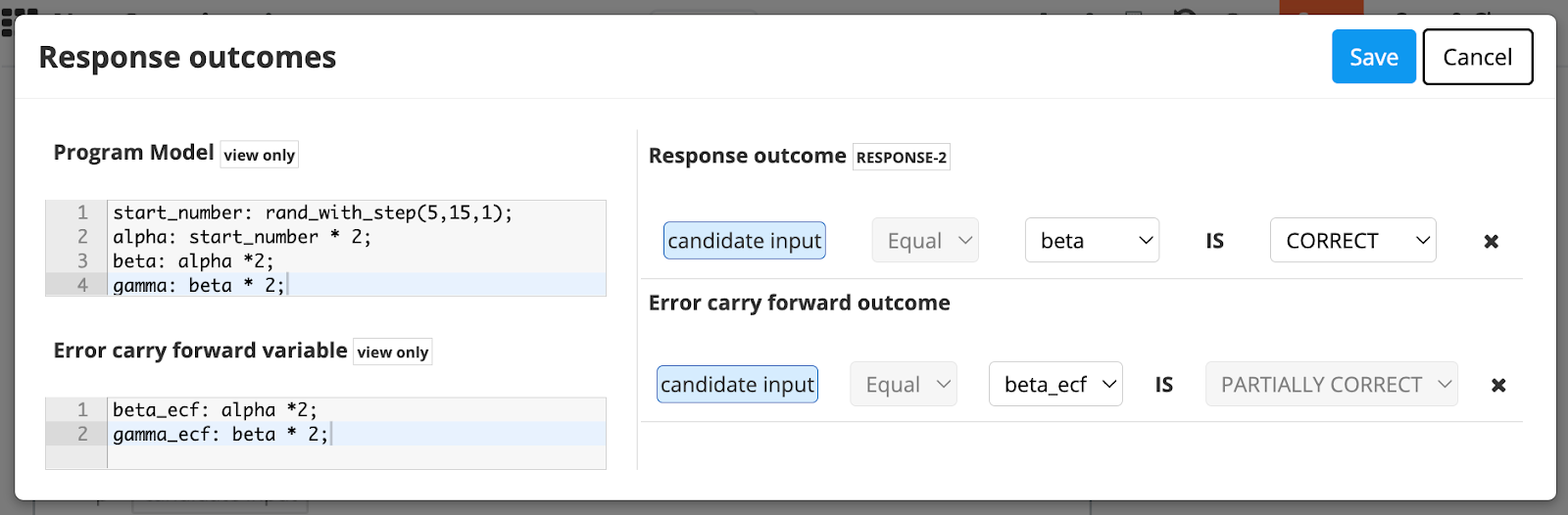Response Outcome RESPONSE-2 “beta”

If the candidate has responded with the incorrect value of “alpha” (RESPONSE-1), but multiplied their incorrect answer by 2 to reach their answer for “beta”, the response to “beta” will be marked as partially correct.

To take this one step further, the third question asks the candidate to calculate “gamma”, which is “beta” multiplied by 2. Above we have defined the ECF variable for “gamma”, and we can enable partially correct marking of “gamma” based on an incorrect answer to “beta”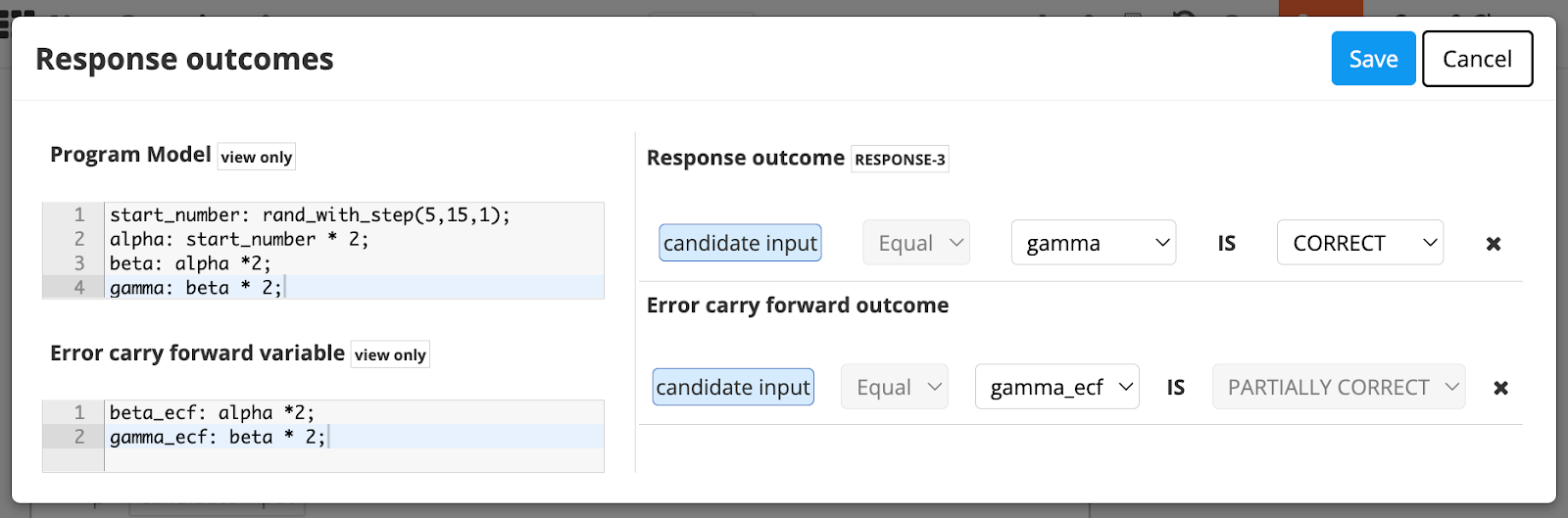Known limitation: With ECF enabled, it is only possible to define one response outcome (in addition to the ECF response outcome) per response.

Tip: You can find many resources on the Internet on Maxima to get you going.

## Author question text

Once the program model is compiled, the Program Variables are available to view under the Program model drop-down.

1. To insert a variable within your question text, click +Insert.
2. You can Insert the following:
• Program Variable
• Candidate Input## Edit response outcome

Response outcomes are the scoring outputs of the Program Model for the candidate input. These outputs represent candidate answers, each can be defined as correct or incorrect and awarded marks accordingly.

### Define the context by choosing a candidate input field

1. To assign a response value to a Program Variable, click [Program Variable].
2. Select the Program Variables drop-down > Choose a Variable.
3. Click Close.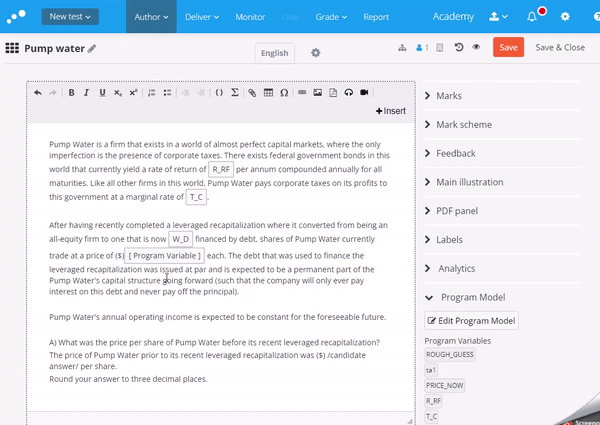### Define the Response outcomes

1. To assign a response value to the Candidate Input Variable, click candidate_input.
2. To add a response outcome, select the Response outcome drop-down > +Add response outcome.
4. Click Save.

### Define feedback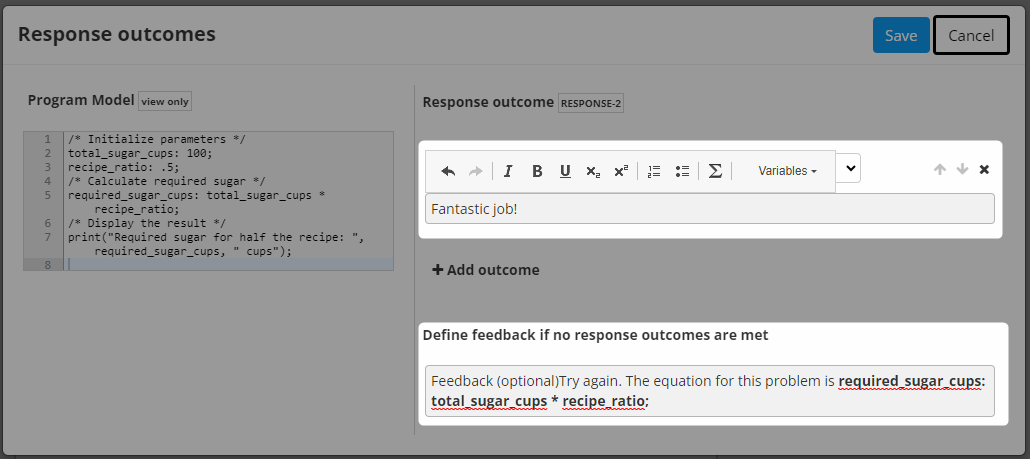You can provide feedback when an outcome is met, or if none of the response outcomes are met. The candidate's response is initially compared to the "correct" response outcome at the top. If the candidate's response does not match, it is then evaluated against the next response outcome in line.

1. To add feedback to a Response outcome, click on the corresponding grey text box and start entering your feedback.
• You can add Rich text to your feedback by selecting the Rich text bar that appears once you've clicked into the grey text box.

There are some known limitations within Feedback:

• General feedback for assessment must be enabled for the feedback on the Response outcome to be available.
• The placeholder text 'Feedback optional', remains visible only in the Authoring view.

### Response outcome options

To determine the Response outcome, you can select from a dropdown list that includes the following options: Comparison Operators, Program Variables, and whether the conclusion is Correct/Incorrect.

• Comparison operators: Equal, Unequal, Greater than, Less than, Abs. Tolerance, Rel. Tolerance
• Program Variables: Variables compiled from the code
• Conclusion: Correct, Incorrect

The actual marks for correct or wrong are set on the question.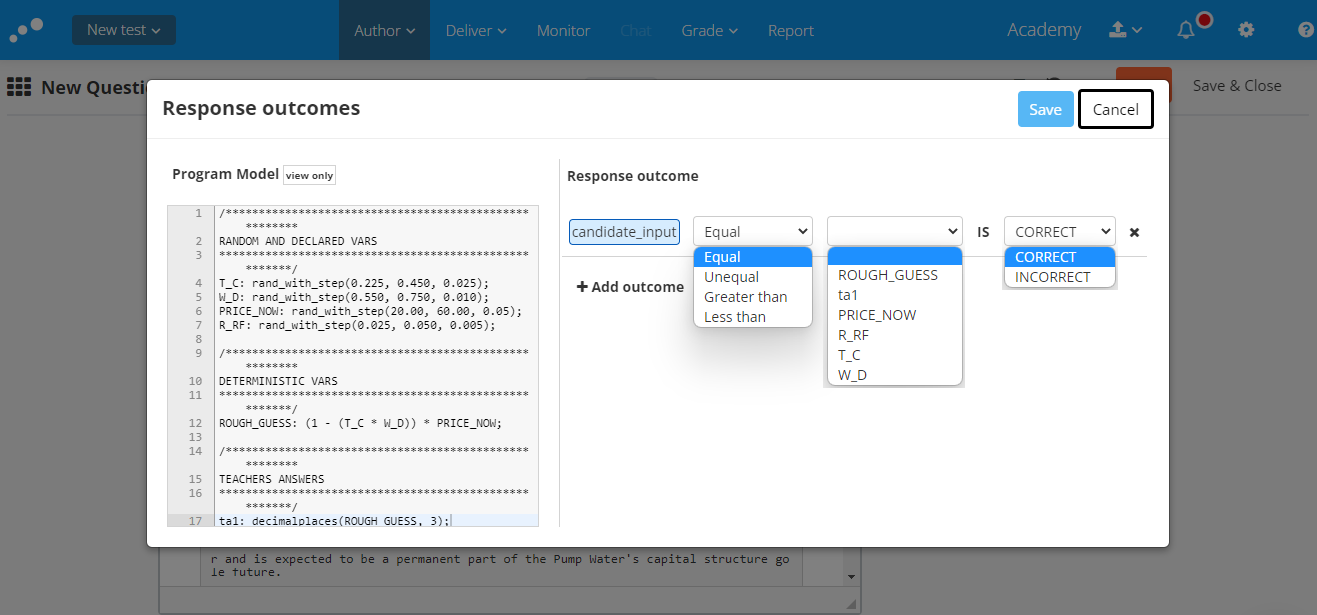## Other Options

You can further customise the response outcome by visiting the Options menu.

1. Select the Options drop-down.
2. From here, you have the following options for input validation which restricts what the candidate can type:
• Expected length
• Expand input field automatically
• Restrict number of characters to input width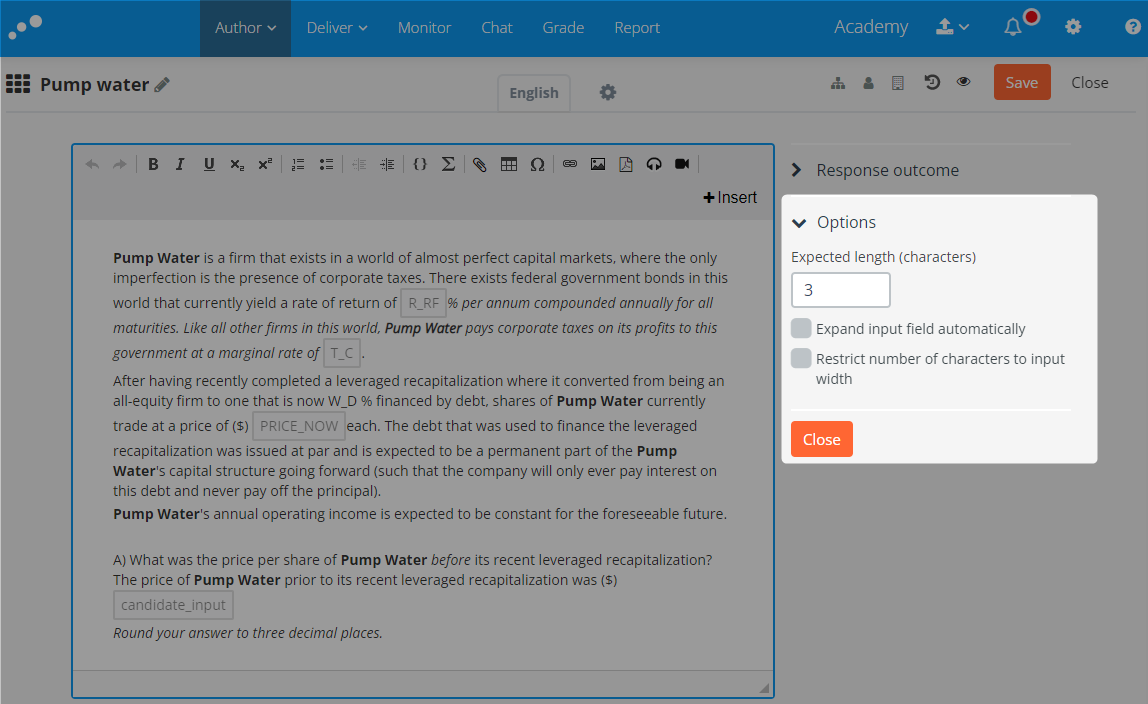Note: The formatting of the Program Variable is not affected by the input field setting. It must for now be defined in the Program Model.

# How to Preview a Numerical Simulation

You can preview your question during the authoring process to see what it looks like for the candidate.

1. Within the Author editor, in the upper right-hand corner, clickPreview.
• Program variables are displayed with their numeric value in the preview pane.
2. Enter an answer within the Candidate input field and click Check answer. To enter a different Candidate input, click Try again
• Wrong answers are given the message Wrong.
• Correct answers are given the message Correct.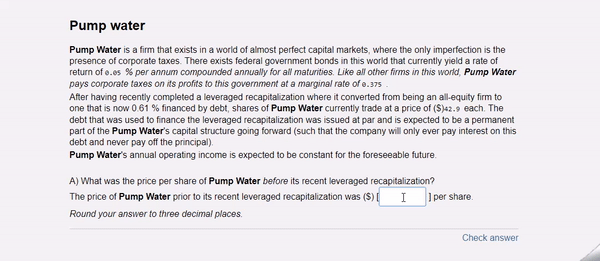# How to Mark a Numerical Simulation

Numerical Simulation is an automatically marked question. Automatically marked questions are automatically marked by Inspera Assessment once the candidate has submitted the test. As the Grader, you do not need to mark an Automatically marked question, unless you intend to override the mark. Automatically marked questions can be found within the Marks dropdown, and are identified with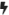Automatically calculated

Numerical simulation question types that contain Error carry forward are rewarded with partial marks.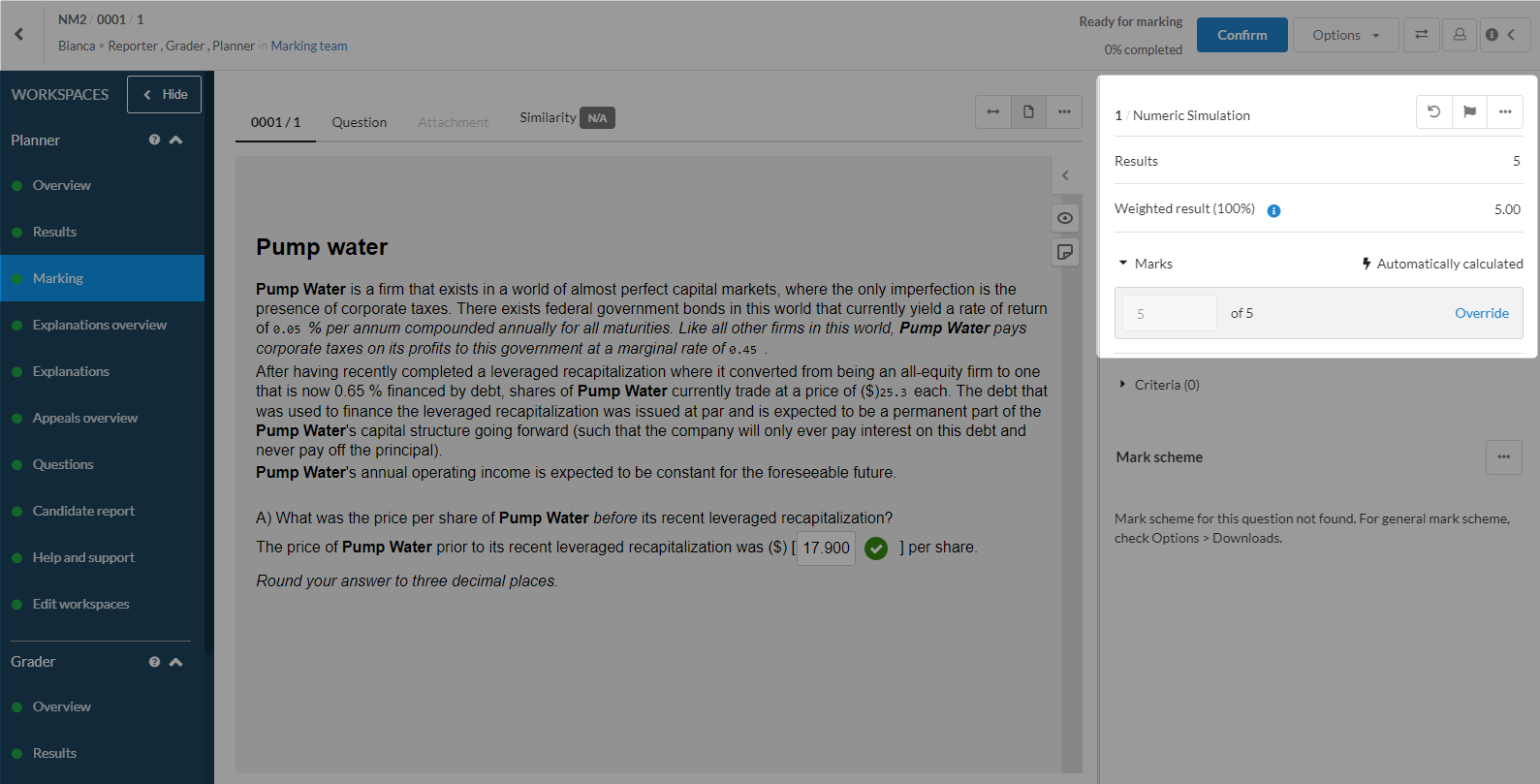## Override an automatically marked question

As a Planner/Grader, you can override the automatically calculated marks.

1. Within the Marks dropdown, Click Override.
2. Within the text field, enter your marks (up to 2 decimal places).
3. Click Apply.

Once you have overridden automatically calculated marks, the status of the question changes to Overridden.